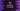# How to find the length of a string in Dart## How to find the length of a string in Dart:

In this post, we will learn how to find the length of a string in Dart. Dart provides one property length in the string class that returns the length of a string. I will show you examples of different strings and finding out the length of these.

### Dart program to find the length of string:

The syntax of length is :

``String.length``

It is an integer value that returns the number of characters in that string. Let’s take a look at the example below:

``````void main() {
String string_1 = 'Hello';
String string_2 = 'Hello world';
String string_3 = '1234';
String string_4 = 'Hello';
String string_5 = '';

print(string_1.length);
print(string_2.length);
print(string_3.length);
print(string_4.length);
print(string_5.length);
}``````

It will print the below output:

``````5
11
4
5
0``````

### Calling length on null:

If we call length on null, it throws one exception:

``````void main() {
String string_1 = null;

print(string_1.length);
}``````

It will give one exception:

``````Unhandled exception:
NoSuchMethodError: The getter 'length' was called on null.